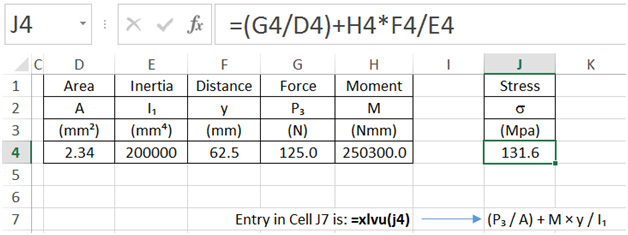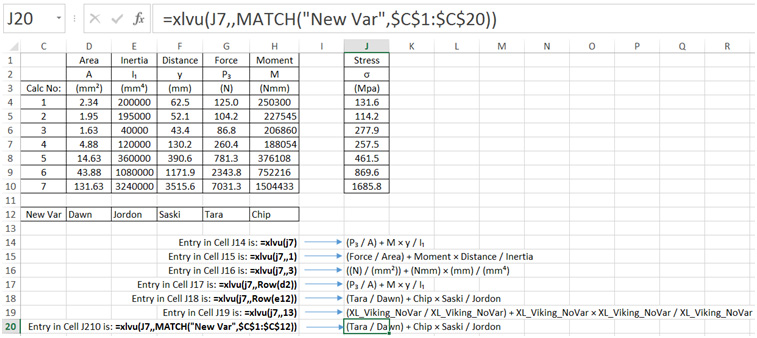# The XLVU Function

## Function for displaying math formula with component variable names – xlvu

=xlvu(CELLREF, Optional Rounder(INTEGER), Optional RowNo(Integer))
This function displays the variable names of a mathematical formula defined in another cell. It updates automatically with a change in the values in the formula or a change in the formula.

Example

A formula (=(G4/D4)+H4*F4/E4) is entered in Cell J4 that references the values in cells D4 to H4. Corresponding variable names are located in cells D2 to H2. Each variable name must be located in the same column as the value it is representing.

The variables of the formula in Cell J4 is shown in the cell where the xlvu function is entered. In the example below, (xlvu(J4)) is entered in Cell J7.How does the xlvu function know to return the variables in Row 2 and not Row 1 or Row 3?

The xlvu function returns the first non-numeric value located above and in the same column as the cell-reference. The xlvu function also ignores cells that contain “(“ or “)” brackets.

But what if I want the xlvu function to show the dimensions (in Row3) or the entire variable names (in Row 1), and I don’t want to re-arrange the location of my variables?

Simply use the second optional parameter to specify the exact row number where the variable you want to show is located. See below for description and examples using the RowNo parameter.

## How to use the Optional Parameters “Rounder” and “RowNo”

Rounder (Integer Value):

The xlvu function is designed to display the variables of the formula. However, in an instance where a numeric value (rather than a CellRef) is entered in the cell that the “xlvu” function is referencing, the rounder parameter can be used to determine the number of decimal places to be displayed. If this parameter is left out (leave as blank), the XL-Viking adaptive number formatting is used.

The adaptive formatting works in the following way:

if Value > 10000000 the number is expressed in Scientific notation
if 10000000 > Value > 100 the number in expressed in standard notation to zero decimal places
if 100 > Value > .001 the number in expressed in standard notation to 3 significant figures
if Value < .001 the number is expressed in Scientific notation

For examples of how the Rounder parameter is used refer to the xln and xlv Functions.

RowNo (Integer value):

By Default the xlvu function returns the first cell above and in the same column as the cell reference that is not a numeric value (and does not contain curly brackets).

In the example below, input data is entered in cells D4:H10. Formulas are in cells J4:J10 that references the input data. Variable names are located above (rows 1:3) as well as below (row 12) the input data. To demonstrate the use of the second optional parameter, the xlvu functions is used in cells J14 to J20. In this example the variables of the formula in cell J7 is shown.Note 1: If there is no variable defined in the row that is specified, the xlvu Function returns “XL_Viking_NoVar” or “xlvikingnovar” to indicate that no variable is defined.

Note 2: The xlvu second Optional Parameter is not used in the above example (hence the double commas), which means any numeric values that are entered in the formula will default to the XL-Viking adaptive number formatting, Ref “Rounder” Parameter.